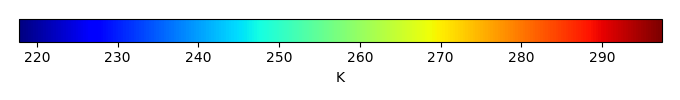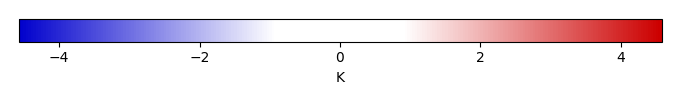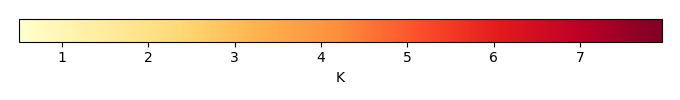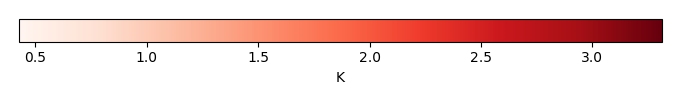# Mean State

Period Mean (original grids) [K]
Model Period Mean (intersection) [K]
Model Period Mean (complement) [K]
Benchmark Period Mean (intersection) [K]
Benchmark Period Mean (complement) [K]
Bias [K]
RMSE [K]
Phase Shift [months]
Bias Score 
RMSE Score 
Seasonal Cycle Score 
Spatial Distribution Score 
Interannual Variability Score 
Overall Score 
Benchmark [-] 293.
CLM5PHSOFF [-] 294. 294. 297. 293. 296. 0.778 1.30 0.646 0.481 0.471 0.926 0.998 0.758 0.684
CLM5PHSON [-] 294. 293. 297. 293. 296. 0.843 1.30 0.638 0.446 0.486 0.928 0.997 0.773 0.686
Period Mean (original grids) [K]
Model Period Mean (intersection) [K]
Model Period Mean (complement) [K]
Benchmark Period Mean (intersection) [K]
Benchmark Period Mean (complement) [K]
Bias [K]
RMSE [K]
Phase Shift [months]
Bias Score 
RMSE Score 
Seasonal Cycle Score 
Spatial Distribution Score 
Interannual Variability Score 
Overall Score 
Benchmark [-] 293.
CLM5PHSOFF [-] 294. 294. 297. 293. 294. 1.15 1.50 0.202 0.622 0.749 0.983 0.986 0.863 0.825
CLM5PHSON [-] 294. 294. 296. 293. 294. 1.17 1.50 0.181 0.620 0.750 0.985 0.987 0.860 0.825
Period Mean (original grids) [K]
Model Period Mean (intersection) [K]
Model Period Mean (complement) [K]
Benchmark Period Mean (intersection) [K]
Benchmark Period Mean (complement) [K]
Bias [K]
RMSE [K]
Phase Shift [months]
Bias Score 
RMSE Score 
Seasonal Cycle Score 
Spatial Distribution Score 
Interannual Variability Score 
Overall Score 
Benchmark [-] 288.
CLM5PHSOFF [-] 290. 289. 294. 288. 293. 1.04 1.39 0.563 0.655 0.785 0.933 1.00 0.840 0.833
CLM5PHSON [-] 289. 289. 293. 288. 293. 1.00 1.36 0.598 0.662 0.786 0.927 1.00 0.839 0.834
Period Mean (original grids) [K]
Model Period Mean (intersection) [K]
Model Period Mean (complement) [K]
Benchmark Period Mean (intersection) [K]
Benchmark Period Mean (complement) [K]
Bias [K]
RMSE [K]
Phase Shift [months]
Bias Score 
RMSE Score 
Seasonal Cycle Score 
Spatial Distribution Score 
Interannual Variability Score 
Overall Score 
Benchmark [-] 256.
CLM5PHSOFF [-] 257. 257. 265. 256. 261. 0.437 1.75 0.0380 0.932 0.918 0.997 0.999 0.907 0.945
CLM5PHSON [-] 256. 256. 264. 256. 261. 0.395 1.75 0.0380 0.932 0.918 0.997 0.999 0.908 0.945
Period Mean (original grids) [K]
Model Period Mean (intersection) [K]
Model Period Mean (complement) [K]
Benchmark Period Mean (intersection) [K]
Benchmark Period Mean (complement) [K]
Bias [K]
RMSE [K]
Phase Shift [months]
Bias Score 
RMSE Score 
Seasonal Cycle Score 
Spatial Distribution Score 
Interannual Variability Score 
Overall Score 
Benchmark [-] 294.
CLM5PHSOFF [-] 296. 295. 297. 294. 295. 1.14 1.62 1.24 0.293 0.499 0.816 0.959 0.828 0.649
CLM5PHSON [-] 295. 295. 297. 294. 295. 1.21 1.66 1.28 0.281 0.505 0.809 0.960 0.814 0.646
Period Mean (original grids) [K]
Model Period Mean (intersection) [K]
Model Period Mean (complement) [K]
Benchmark Period Mean (intersection) [K]
Benchmark Period Mean (complement) [K]
Bias [K]
RMSE [K]
Phase Shift [months]
Bias Score 
RMSE Score 
Seasonal Cycle Score 
Spatial Distribution Score 
Interannual Variability Score 
Overall Score 
Benchmark [-] 265.
CLM5PHSOFF [-] 265. 265. 272. 265. 269. -0.138 1.13 0.0181 0.947 0.943 0.999 0.997 0.965 0.966
CLM5PHSON [-] 265. 265. 272. 265. 269. -0.198 1.13 0.0191 0.945 0.945 0.999 0.997 0.965 0.966
Period Mean (original grids) [K]
Model Period Mean (intersection) [K]
Model Period Mean (complement) [K]
Benchmark Period Mean (intersection) [K]
Benchmark Period Mean (complement) [K]
Bias [K]
RMSE [K]
Phase Shift [months]
Bias Score 
RMSE Score 
Seasonal Cycle Score 
Spatial Distribution Score 
Interannual Variability Score 
Overall Score 
Benchmark [-] 274.
CLM5PHSOFF [-] 275. 275. 286. 274. 280. 0.732 1.56 0.0151 0.879 0.924 0.999 1.00 0.928 0.942
CLM5PHSON [-] 274. 274. 285. 274. 280. 0.670 1.54 0.0163 0.881 0.923 0.999 0.999 0.928 0.942
Period Mean (original grids) [K]
Model Period Mean (intersection) [K]
Model Period Mean (complement) [K]
Benchmark Period Mean (intersection) [K]
Benchmark Period Mean (complement) [K]
Bias [K]
RMSE [K]
Phase Shift [months]
Bias Score 
RMSE Score 
Seasonal Cycle Score 
Spatial Distribution Score 
Interannual Variability Score 
Overall Score 
Benchmark [-] 291.
CLM5PHSOFF [-] 292. 292. 297. 291. 296. 0.795 1.53 0.241 0.712 0.845 0.980 0.999 0.926 0.884
CLM5PHSON [-] 291. 291. 297. 291. 296. 0.736 1.51 0.255 0.717 0.846 0.978 0.999 0.929 0.886
Period Mean (original grids) [K]
Model Period Mean (intersection) [K]
Model Period Mean (complement) [K]
Benchmark Period Mean (intersection) [K]
Benchmark Period Mean (complement) [K]
Bias [K]
RMSE [K]
Phase Shift [months]
Bias Score 
RMSE Score 
Seasonal Cycle Score 
Spatial Distribution Score 
Interannual Variability Score 
Overall Score 
Benchmark [-] 259.
CLM5PHSOFF [-] 259. 259. 265. 259. 260. -0.180 1.47 0.0268 0.956 0.923 0.998 1.00 0.920 0.953
CLM5PHSON [-] 259. 259. 263. 259. 260. -0.224 1.48 0.0263 0.954 0.923 0.998 1.00 0.921 0.953
Period Mean (original grids) [K]
Model Period Mean (intersection) [K]
Model Period Mean (complement) [K]
Benchmark Period Mean (intersection) [K]
Benchmark Period Mean (complement) [K]
Bias [K]
RMSE [K]
Phase Shift [months]
Bias Score 
RMSE Score 
Seasonal Cycle Score 
Spatial Distribution Score 
Interannual Variability Score 
Overall Score 
Benchmark [-] 287.
CLM5PHSOFF [-] 289. 289. 290. 287. 289. 1.17 1.35 0.134 0.770 0.888 0.991 1.00 0.942 0.913
CLM5PHSON [-] 289. 289. 290. 287. 289. 1.16 1.34 0.138 0.770 0.891 0.991 1.00 0.943 0.914
Period Mean (original grids) [K]
Model Period Mean (intersection) [K]
Model Period Mean (complement) [K]
Benchmark Period Mean (intersection) [K]
Benchmark Period Mean (complement) [K]
Bias [K]
RMSE [K]
Phase Shift [months]
Bias Score 
RMSE Score 
Seasonal Cycle Score 
Spatial Distribution Score 
Interannual Variability Score 
Overall Score 
Benchmark [-] 277.
CLM5PHSOFF [-] 278. 277. 282. 277. 281. 0.100 1.14 0.216 0.859 0.907 0.985 0.993 0.941 0.932
CLM5PHSON [-] 277. 277. 280. 277. 281. 0.00878 1.14 0.219 0.859 0.905 0.985 0.994 0.942 0.932
Period Mean (original grids) [K]
Model Period Mean (intersection) [K]
Model Period Mean (complement) [K]
Benchmark Period Mean (intersection) [K]
Benchmark Period Mean (complement) [K]
Bias [K]
RMSE [K]
Phase Shift [months]
Bias Score 
RMSE Score 
Seasonal Cycle Score 
Spatial Distribution Score 
Interannual Variability Score 
Overall Score 
Benchmark [-] 281.
CLM5PHSOFF [-] 278. 281. 257. 280. 287. 0.718 1.47 0.268 0.759 0.833 0.968 0.999 0.909 0.884
CLM5PHSON [-] 277. 281. 244. 280. 287. 0.686 1.46 0.269 0.759 0.835 0.968 0.999 0.909 0.884
Period Mean (original grids) [K]
Model Period Mean (intersection) [K]
Model Period Mean (complement) [K]
Benchmark Period Mean (intersection) [K]
Benchmark Period Mean (complement) [K]
Bias [K]
RMSE [K]
Phase Shift [months]
Bias Score 
RMSE Score 
Seasonal Cycle Score 
Spatial Distribution Score 
Interannual Variability Score 
Overall Score 
Benchmark [-] 289.
CLM5PHSOFF [-] 289. 289. 293. 288. 292. 0.668 1.30 0.235 0.850 0.883 0.982 1.00 0.908 0.918
CLM5PHSON [-] 289. 289. 292. 288. 292. 0.623 1.29 0.238 0.852 0.882 0.982 1.00 0.909 0.918
Period Mean (original grids) [K]
Model Period Mean (intersection) [K]
Model Period Mean (complement) [K]
Benchmark Period Mean (intersection) [K]
Benchmark Period Mean (complement) [K]
Bias [K]
RMSE [K]
Phase Shift [months]
Bias Score 
RMSE Score 
Seasonal Cycle Score 
Spatial Distribution Score 
Interannual Variability Score 
Overall Score 
Benchmark [-] 260.
CLM5PHSOFF [-] 261. 260. 264. 260. 262. 0.424 1.52 0.0476 0.938 0.915 0.997 0.998 0.920 0.947
CLM5PHSON [-] 260. 260. 262. 260. 262. 0.385 1.52 0.0456 0.938 0.915 0.997 0.998 0.920 0.947
Period Mean (original grids) [K]
Model Period Mean (intersection) [K]
Model Period Mean (complement) [K]
Benchmark Period Mean (intersection) [K]
Benchmark Period Mean (complement) [K]
Bias [K]
RMSE [K]
Phase Shift [months]
Bias Score 
RMSE Score 
Seasonal Cycle Score 
Spatial Distribution Score 
Interannual Variability Score 
Overall Score 
Benchmark [-] 289.
CLM5PHSOFF [-] 291. 290. 295. 289. 293. 1.41 2.01 0.355 0.567 0.765 0.964 0.999 0.907 0.828
CLM5PHSON [-] 289. 289. 294. 289. 293. 1.40 2.00 0.369 0.568 0.766 0.961 0.999 0.905 0.828
Period Mean (original grids) [K]
Model Period Mean (intersection) [K]
Model Period Mean (complement) [K]
Benchmark Period Mean (intersection) [K]
Benchmark Period Mean (complement) [K]
Bias [K]
RMSE [K]
Phase Shift [months]
Bias Score 
RMSE Score 
Seasonal Cycle Score 
Spatial Distribution Score 
Interannual Variability Score 
Overall Score 
Benchmark [-] 280.
CLM5PHSOFF [-] 281. 281. 289. 280. 287. 1.13 1.32 0.0279 0.870 0.938 0.998 1.00 0.971 0.952
CLM5PHSON [-] 281. 281. 289. 280. 287. 1.06 1.27 0.0255 0.876 0.936 0.998 1.00 0.972 0.953
Period Mean (original grids) [K]
Model Period Mean (intersection) [K]
Model Period Mean (complement) [K]
Benchmark Period Mean (intersection) [K]
Benchmark Period Mean (complement) [K]
Bias [K]
RMSE [K]
Phase Shift [months]
Bias Score 
RMSE Score 
Seasonal Cycle Score 
Spatial Distribution Score 
Interannual Variability Score 
Overall Score 
Benchmark [-] 268.
CLM5PHSOFF [-] 269. 269. 276. 268. 274. 0.498 1.18 0.0384 0.920 0.924 0.997 0.997 0.950 0.952
CLM5PHSON [-] 268. 268. 274. 268. 274. 0.439 1.14 0.0361 0.922 0.926 0.998 0.997 0.952 0.953
Period Mean (original grids) [K]
Model Period Mean (intersection) [K]
Model Period Mean (complement) [K]
Benchmark Period Mean (intersection) [K]
Benchmark Period Mean (complement) [K]
Bias [K]
RMSE [K]
Phase Shift [months]
Bias Score 
RMSE Score 
Seasonal Cycle Score 
Spatial Distribution Score 
Interannual Variability Score 
Overall Score 
Benchmark [-] 276.
CLM5PHSOFF [-] 277. 277. 284. 276. 280. 1.56 2.02 0.0424 0.785 0.890 0.997 0.998 0.938 0.916
CLM5PHSON [-] 277. 277. 283. 276. 280. 1.52 2.00 0.0516 0.787 0.891 0.997 0.998 0.943 0.918
Period Mean (original grids) [K]
Model Period Mean (intersection) [K]
Model Period Mean (complement) [K]
Benchmark Period Mean (intersection) [K]
Benchmark Period Mean (complement) [K]
Bias [K]
RMSE [K]
Phase Shift [months]
Bias Score 
RMSE Score 
Seasonal Cycle Score 
Spatial Distribution Score 
Interannual Variability Score 
Overall Score 
Benchmark [-] 294.
CLM5PHSOFF [-] 295. 295. 297. 294. 294. 0.871 1.36 1.13 0.416 0.538 0.828 0.996 0.881 0.699
CLM5PHSON [-] 295. 295. 297. 294. 294. 0.925 1.38 1.11 0.399 0.552 0.833 0.996 0.871 0.700
Period Mean (original grids) [K]
Model Period Mean (intersection) [K]
Model Period Mean (complement) [K]
Benchmark Period Mean (intersection) [K]
Benchmark Period Mean (complement) [K]
Bias [K]
RMSE [K]
Phase Shift [months]
Bias Score 
RMSE Score 
Seasonal Cycle Score 
Spatial Distribution Score 
Interannual Variability Score 
Overall Score 
Benchmark [-] 290.
CLM5PHSOFF [-] 291. 291. 293. 290. 288. 1.10 1.34 0.512 0.629 0.795 0.931 1.00 0.937 0.848
CLM5PHSON [-] 291. 291. 293. 290. 288. 1.06 1.31 0.478 0.637 0.798 0.938 1.00 0.935 0.851
Period Mean (original grids) [K]
Model Period Mean (intersection) [K]
Model Period Mean (complement) [K]
Benchmark Period Mean (intersection) [K]
Benchmark Period Mean (complement) [K]
Bias [K]
RMSE [K]
Phase Shift [months]
Bias Score 
RMSE Score 
Seasonal Cycle Score 
Spatial Distribution Score 
Interannual Variability Score 
Overall Score 
Benchmark [-] 278.
CLM5PHSOFF [-] 279. 279. 285. 278. 281. 1.29 2.21 0.117 0.590 0.794 0.990 1.00 0.922 0.848
CLM5PHSON [-] 279. 278. 284. 278. 281. 1.24 2.18 0.122 0.596 0.793 0.989 1.00 0.922 0.849

# Temporally integrated period mean

BENCHMARK MEANMODEL MEANBIASBIAS SCORERMSERMSE SCOREBENCHMARK INTERANNUAL VARIABILITYMODEL INTERANNUAL VARIABILITYINTERANNUAL VARIABILITY SCOREBENCHMARK MAX MONTHMODEL MAX MONTHDIFFERENCE IN MAX MONTHSEASONAL CYCLE SCORESPATIAL TAYLOR DIAGRAMMODEL COLORS# Spatially integrated regional mean

MODEL COLORSREGIONAL MEANANNUAL CYCLEMONTHLY ANOMALYANNUAL CYCLE# All Models

BenchmarkCLM5PHSOFFCLM5PHSON# Data Information

creation_date: Fri Feb 8 14:17:30 PST 2019

history: 2018-01-15: download from https://crudata.uea.ac.uk/cru/data/hrg/cru_ts_4.01/cruts.1709081022.v4.01/ 2019-02-06: converted and created to netCDF4 for ILAMB package

original_resolution: 0.5x0.5

resolution: 0.5x0.5

original_frequency: mon

frequency: mon

references: Harris et al., 2014, Int. J. Climatol., doi:10.1002/joc.3711

institution: Original Data held at British Atmospheric Data Centre, RAL, UK.

source: Run ID = 1709081022. Data generated from:TMN derived from gridded TMP & DTR absolutes, DTR in turn derived from tmn.1708171135.dtb, tmx.1708171215.dtb

title: CRU TS4.01 Minimum Temperature

Conventions: CF-1.7